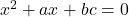1. Show that each pair of following equations has a common root
1.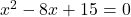and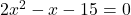2.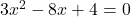and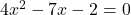2. Find the value of p so that each pair of the equations may have one root common
1.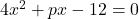and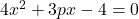2.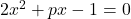and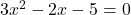3. If the quadratic equations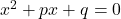and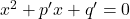have common roots show that it must be either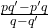or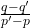4. If the quadratic equationsand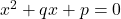have common roots show that it must be eitheror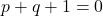5. If the quadratic equations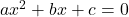and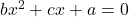have common roots show that it must be either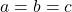or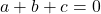6. Prove that if the equations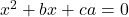and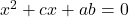have a common root, their other root will satisfy